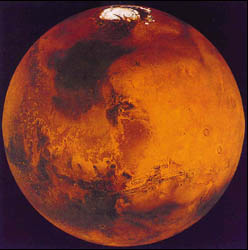﻿ Weight On Mars Calculator ﻿# Weight on Mars Calculator### How Much Would You Or Any Object Weigh On Mars?Enter Weight: Any unit grams (g) kilograms (kg) pounds (lbs)

This Weight on Mars Conversion Calculator calculates your weight or the weight that any object would be if on Mars.

To use this calculator, a user just enters his or her weight and clicks the 'Calculate' button, and his/her weight will automatically be computed and shown below. The weight can be entered in any units and the resultant answer will be in the same units which the user has input. Thus, for example, if a user enters 170lbs, the resultant answer of the weight in Mars would be 64.31lbs. The units will always match. Just for the sake of clarity, a user can select the units he wants the answer to appear in, whether grams, kilograms, or pounds, and that unit will show in the answer.

This calculates the weight on mars from the weight of the object on earth. Being that it takes the weight of an object on earth and converts it to Martian weight, the formula is Weight on Mars= (Weight on Earth/9.81m/s2) * 3.711m/s2. To find the Martian weight, we divide the weight on earth by the earth's force of gravity, which is 9.81m/s2. This calculates the mass of the object. Once we have the object's mass, we can find the weight by multiplying it by the gravitational force, which it is subject to. Being that Mars has a gravitational force of 3.711m/s2, we multiply the object's mass by this quanitity to calculate an object's weight on mars.

So an object or person on Mars would weigh 37.83% its weight on earth. Therefore, a person would be much lighter on mars. Conversely, a person is 62.17% heavier on earth than on Mars.

What causes the differences in weight between the various planets? The answer is the gravitational force which a planet is subject to. Earth has much more mass than Mars. Therefore, the sun pulls on it with greater force, because of the greater mass. You probably are familiar that gravity is a downward force. It pushes you back to the Earth's surface. This is why when you jump, you come back down. Being that the earth has greater gravitational force, it pulls object downward with greater force. This translates into greater weight.

I did this calculator out of inspiration from the Mars Rover Curiosity landing from NASA. I just thought being that we're sending rovers out there, it may not be too far from a manned mission to Mars. I was just thinking about the weight of objects on mars. How much do they weigh? Once I googled Mar's gravitational force, I could figure out what any object would weigh on Mars.

If you want to calculate the weight of all of the planets simultaneously, see our Weight on Planets Calculator.

Related Resources

﻿